# Lions Weekly: March 22, 2023# A Note From Mr. Sandefur

Lion Family,

Welcome back! I pray that this past week was such a blessing for you and your family and that you were able to make quality memories with friends and family. We have officially reached the final stretch of the academic year and are preparing for the mad dash to graduation. Please join me in praying specifically for our seniors and their parents as the next few weeks will go very quickly and be meaningful for them during this special season.

I had the distinct pleasure of traveling with twenty of our students and parents to Europe over spring break and am grateful to report that our students had an incredible learning experience. One of my favorite aspects of The King’s Academy is the global mindset that exposes students to the world we live in and how the Creator is glorified through his creation.

Upon returning from Europe, Lee Smith and I were able to attend the Tennessee Baptist Mission Board’s retreat for Partner Ministries. This retreat allowed us to share the good news of all that is occurring on our campus and through the reach of this incredible ministry. Please join me in continuing to pray for our partnership with the TBMB as we partner together to develop servant leaders that will change the world for Christ.

Jeremy Sandefur

 table div table+table+table+table div table{width:100%;padding:0}table div table+table+table+table div table img{width:96.23%;padding:0;float:none}table div table+table+table+table div table td{width:100%;padding:0 1.88% 18px}/* styles */table div table+table+table+table+table+table div table{width:100%;padding:0}table div table+table+table+table+table+table div table img{width:96.23%;padding:0;float:none}table div table+table+table+table+table+table div table td{width:100%;padding:0 1.88% 18px}/* styles */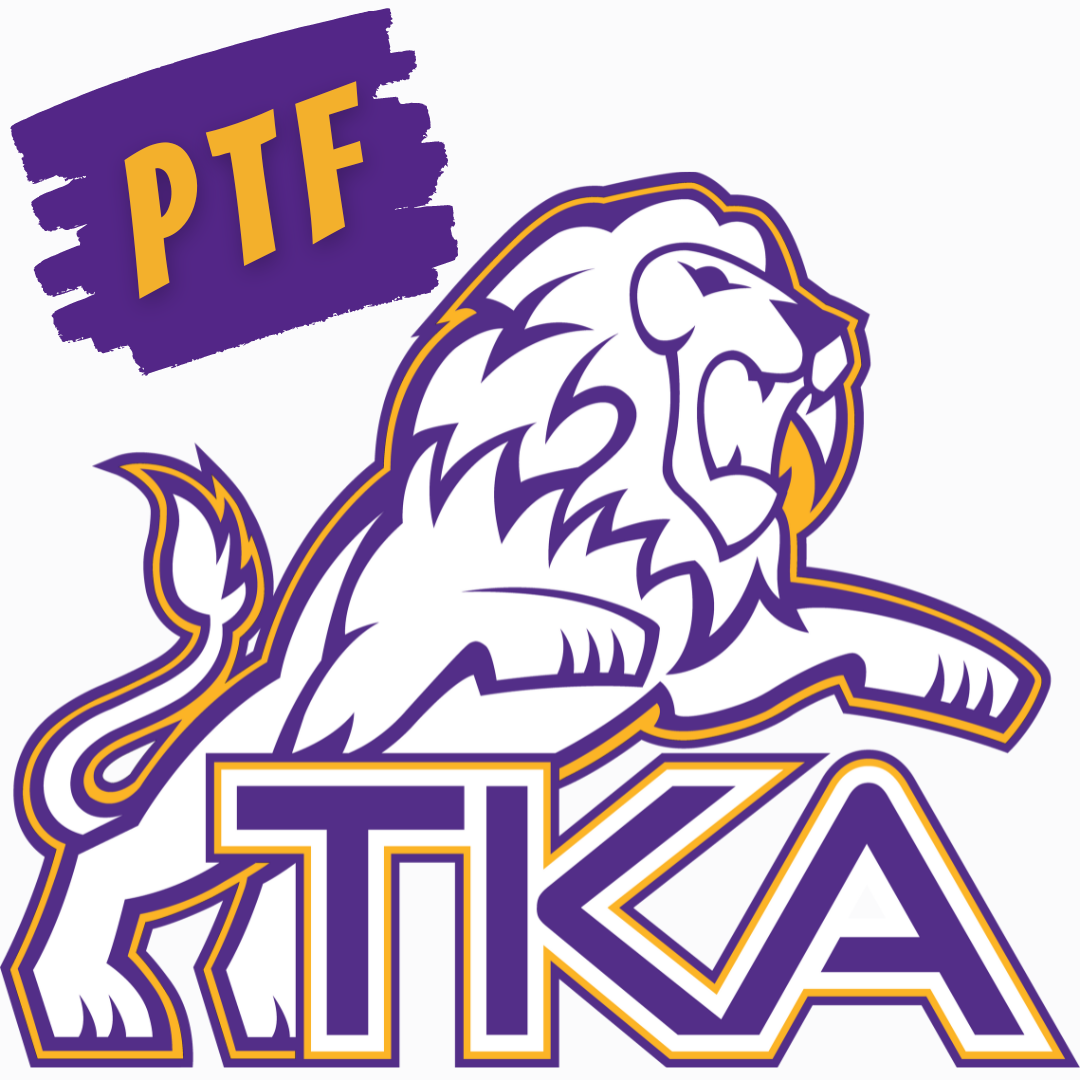# PTF Meeting

Our next PTF meeting takes place tomorrow at 3:15 in Brandes! We hope to see everyone at the meeting!

 table div table+table+table+table+table+table+table+table div table{width:100%;padding:0}table div table+table+table+table+table+table+table+table div table img{width:96.23%;padding:0;float:none}table div table+table+table+table+table+table+table+table div table td{width:100%;padding:0 1.88% 18px}/* styles */# SGA & BETA

Our High School SGA and BETA are currently taking gift card donations for Isaiah 117 House. The gift card drive will run from now until Wednesday, March 22. Gift cards can be dropped off in the front offices or purchased through SCRIP.

## How to donate:

1. Drop off gift cards in the front office.
2. Purchase gift cards through SCRIP.
 1 Drop off gift cards in the front office.
 2 Purchase gift cards through SCRIP.

## Scrip Donation Information:

2. Text Cindy Tucker at 865-804-1924 to let her know your order is for BETA.
3. If you are enrolling in SCRIP for the first time, use this code for enrollment: FC75F12F26641
 2 Text Cindy Tucker at 865-804-1924 to let her know your order is for BETA.
 3 If you are enrolling in SCRIP for the first time, use this code for enrollment: FC75F12F26641

Donations and online orders are due by Wednesday, March 22

 table div table+table+table+table+table+table+table+table+table+table+table div table{width:100%;padding:0}table div table+table+table+table+table+table+table+table+table+table+table div table img{width:96.23%;padding:0;float:none}table div table+table+table+table+table+table+table+table+table+table+table div table td{width:100%;padding:0 1.88% 18px}/* styles */# We're Hiring!

TKA has an employment opportunity available in food services.

Food Services Employee: TKA is seeking an employee to faithfully serve in our food services department. Prior food service experience is preferred. Please email resumes to HR@thekingsacademy.net or call 865-573-8321.

 table div table+table+table+table+table+table+table+table+table+table+table+table+table+table div table{width:100%;padding:0}table div table+table+table+table+table+table+table+table+table+table+table+table+table+table div table img{width:96.23%;padding:0;float:none}table div table+table+table+table+table+table+table+table+table+table+table+table+table+table div table td{width:100%;padding:0 1.88% 18px}/* styles */# Prom Information

DATE: April 1, 2023
PLACE: Capitol Theatre, Maryville, TN
TIME: 6:00 pm - 10:30 pm

# Ticket Information

Tickets for this year's High School Prom are on sale now!

COST: \$55 per person from March 18-March 31

## How to purchase tickets:

▪ The student must enter the promotional code: TKA37865
▪ Maximum 2 tickets per order
▪ After selecting the number of tickets to purchase, you must enter Additional Information on the 2nd page before completing the purchase.
 ▪ The student must enter the promotional code: TKA37865
 ▪ Maximum 2 tickets per order
 ▪ After selecting the number of tickets to purchase, you must enter Additional Information on the 2nd page before completing the purchase.

# Prom Details

DATE: April 1, 2023
PLACE: Capitol Theatre, Maryville, TN
TIME: 6:00 pm - 10:30 pm
COST: \$50 per person through March 17/\$55 per person March 18-March 31.

Desserts/Light Refreshments will be served.

OUTSIDE GUESTS:
ALL outside guests must be approved. An outside guest form can be found in Mrs. Griffin's office or by using the link below.

DRESS CODE REQUIREMENTS:
Girls - Prom and homecoming dresses must be viewed on the individual in advance for suitable fitting - no exceptions. Skirts of the dresses may not be sheer (see through) material. “Dollar Bill” test will be used for the length. Length should be no shorter than width of dollar bill above knee (approximately two inches). Neckline and backline must be modest (backline must be above the natural waistline). Spaghetti straps and strapless styles are acceptable, if modest. The midriff should always remain covered. No open sides or sheer panels. No immodest tight dresses are allowed. Viewing dates will be provided for both homecoming and prom.

Boys - Suit & Tie or Tuxedo, Dress Shoes

PROM DRESS CHECKS WILL BE:
March 23 3:15-4:00
Or via email to lwallace@thekingsacademy.net and kpolk@thekingsacademy.net - send pics of front and back full length of dress in good lighting.

Remember, all attire must adhere to dress code.

## Contact Jenny Cook via text 865-387-3468 with any prom related questions.

 table div table+table+table+table+table+table+table+table+table+table+table+table+table+table+table+table+table+table+table+table div table{width:100%;padding:0}table div table+table+table+table+table+table+table+table+table+table+table+table+table+table+table+table+table+table+table+table div table img{width:96.23%;padding:0;float:none}table div table+table+table+table+table+table+table+table+table+table+table+table+table+table+table+table+table+table+table+table div table td{width:100%;padding:0 1.88% 18px}/* styles */table div table+table+table+table+table+table+table+table+table+table+table+table+table+table+table+table+table+table+table+table+table+table div table{width:100%;padding:0}table div table+table+table+table+table+table+table+table+table+table+table+table+table+table+table+table+table+table+table+table+table+table div table img{width:96.23%;padding:0;float:none}table div table+table+table+table+table+table+table+table+table+table+table+table+table+table+table+table+table+table+table+table+table+table div table td{width:100%;padding:0 1.88% 18px}/* styles */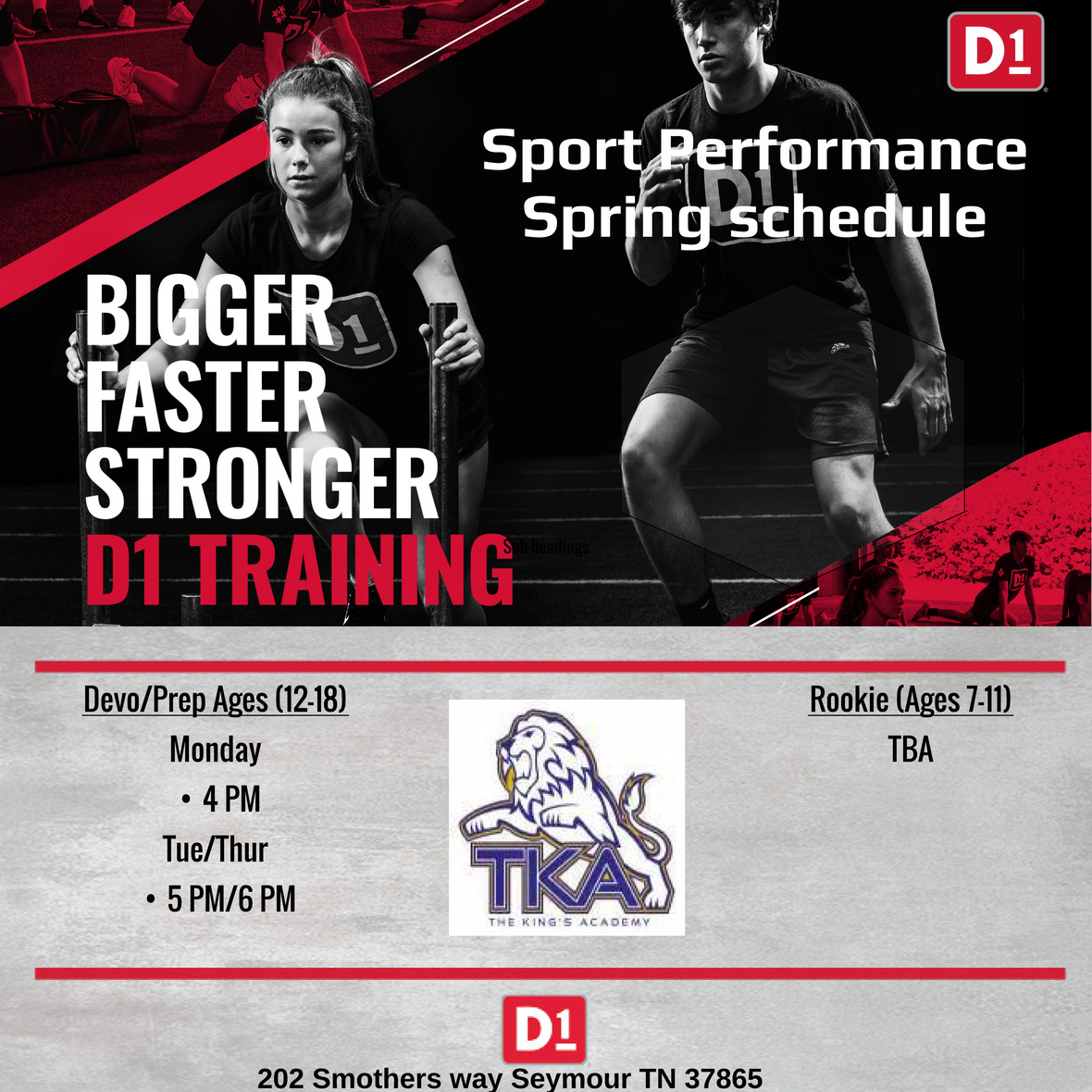# D1 Training

Middle/High school athletes can come train outside of their team training hours at the listed times.

For any additional information, parents can contact Mr. Melvin Jones.

Melvin Jones
D1 Sevierville
865-719-9421
melvin.jones@d1training.com

 table div table+table+table+table+table+table+table+table+table+table+table+table+table+table+table+table+table+table+table+table+table+table+table+table div table{width:100%;padding:0}table div table+table+table+table+table+table+table+table+table+table+table+table+table+table+table+table+table+table+table+table+table+table+table+table div table img{width:96.23%;padding:0;float:none}table div table+table+table+table+table+table+table+table+table+table+table+table+table+table+table+table+table+table+table+table+table+table+table+table div table td{width:100%;padding:0 1.88% 18px}/* styles */# 2022-23 Yearbooks

2022-23 yearbooks are now on sale! Scan this QR code to visit the site to purchase yours today. For any questions regarding yearbooks, contact Mr. Josh Smith at jsmith@thekingsacademy.net.

 table div table+table+table+table+table+table+table+table+table+table+table+table+table+table+table+table+table+table+table+table+table+table+table+table+table+table div table{width:100%;padding:0}table div table+table+table+table+table+table+table+table+table+table+table+table+table+table+table+table+table+table+table+table+table+table+table+table+table+table div table img{width:96.23%;padding:0;float:none}table div table+table+table+table+table+table+table+table+table+table+table+table+table+table+table+table+table+table+table+table+table+table+table+table+table+table div table td{width:100%;padding:0 1.88% 18px}/* styles */# Middle School Golf Update

Our middle school golf teams are off to a great start! The Lady Lions won their match Monday against Northview, and Camdyn Cole for placed 1st individually! Way to go Lions!

 table div table+table+table+table+table+table+table+table+table+table+table+table+table+table+table+table+table+table+table+table+table+table+table+table+table+table+table+table div table{width:100%;padding:0}table div table+table+table+table+table+table+table+table+table+table+table+table+table+table+table+table+table+table+table+table+table+table+table+table+table+table+table+table div table img{width:96.23%;padding:0;float:none}table div table+table+table+table+table+table+table+table+table+table+table+table+table+table+table+table+table+table+table+table+table+table+table+table+table+table+table+table div table td{width:100%;padding:0 1.88% 18px}/* styles */# Weekly Sports Schedule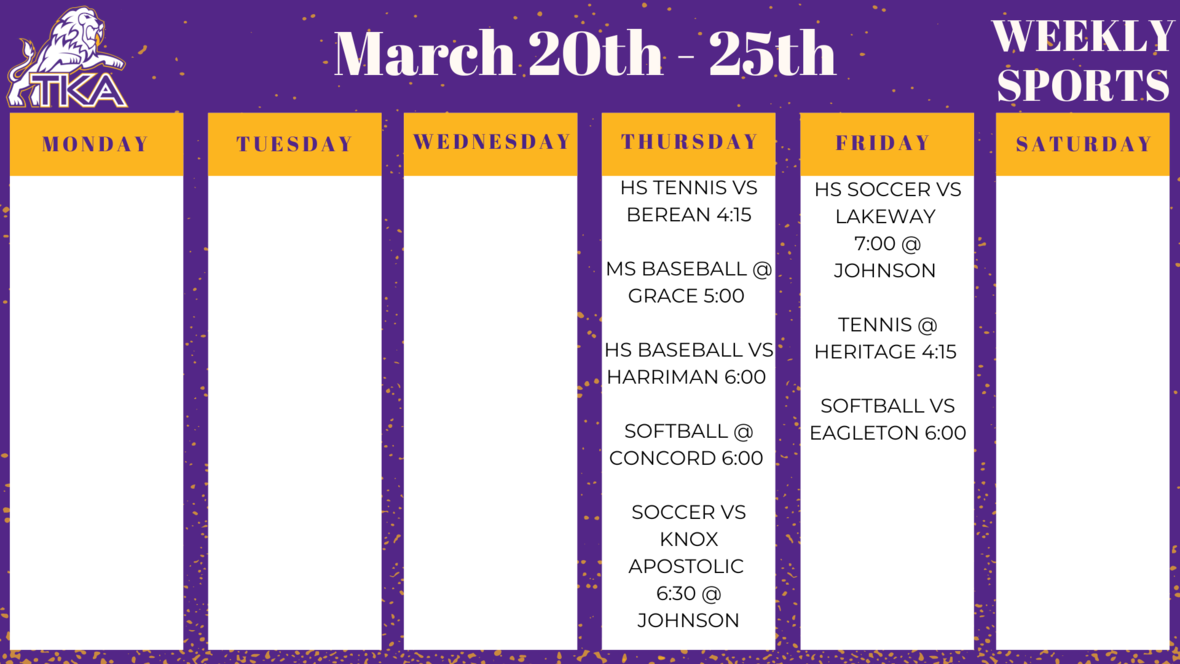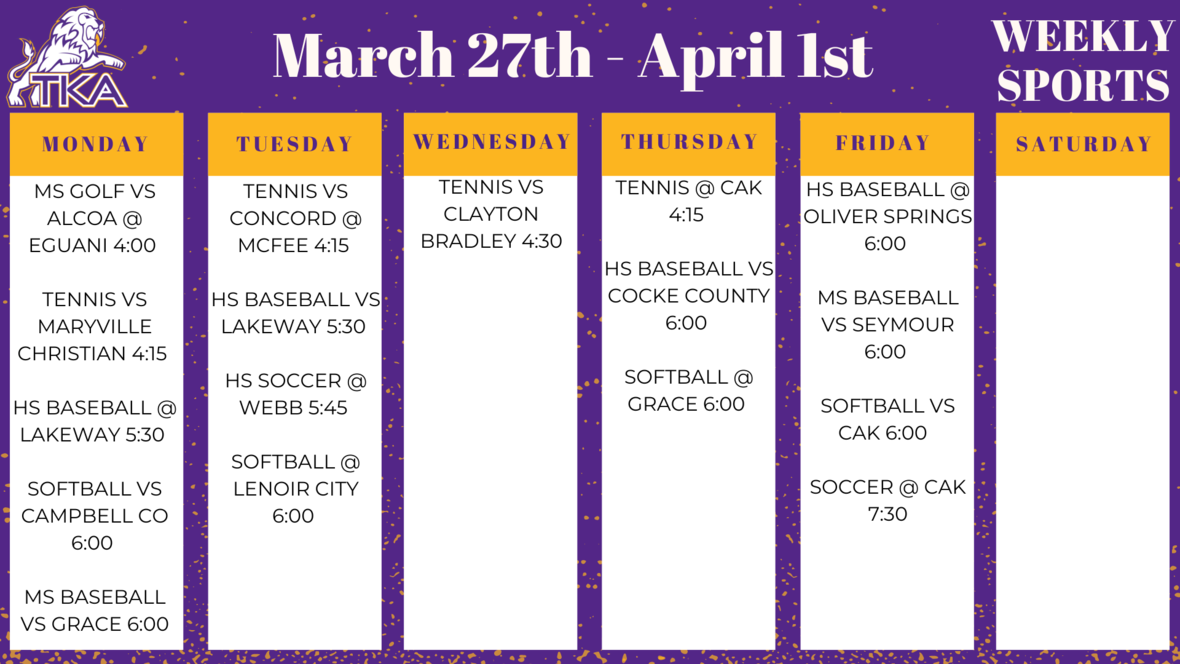table div table+table+table+table+table+table+table+table+table+table+table+table+table+table+table+table+table+table+table+table+table+table+table+table+table+table+table+table+table+table+table+table div table{width:100%;padding:0}table div table+table+table+table+table+table+table+table+table+table+table+table+table+table+table+table+table+table+table+table+table+table+table+table+table+table+table+table+table+table+table+table div table img{width:96.23%;padding:0;float:none}table div table+table+table+table+table+table+table+table+table+table+table+table+table+table+table+table+table+table+table+table+table+table+table+table+table+table+table+table+table+table+table+table div table td{width:100%;padding:0 1.88% 18px}/* styles */# Important information for the 2022-2023 School Year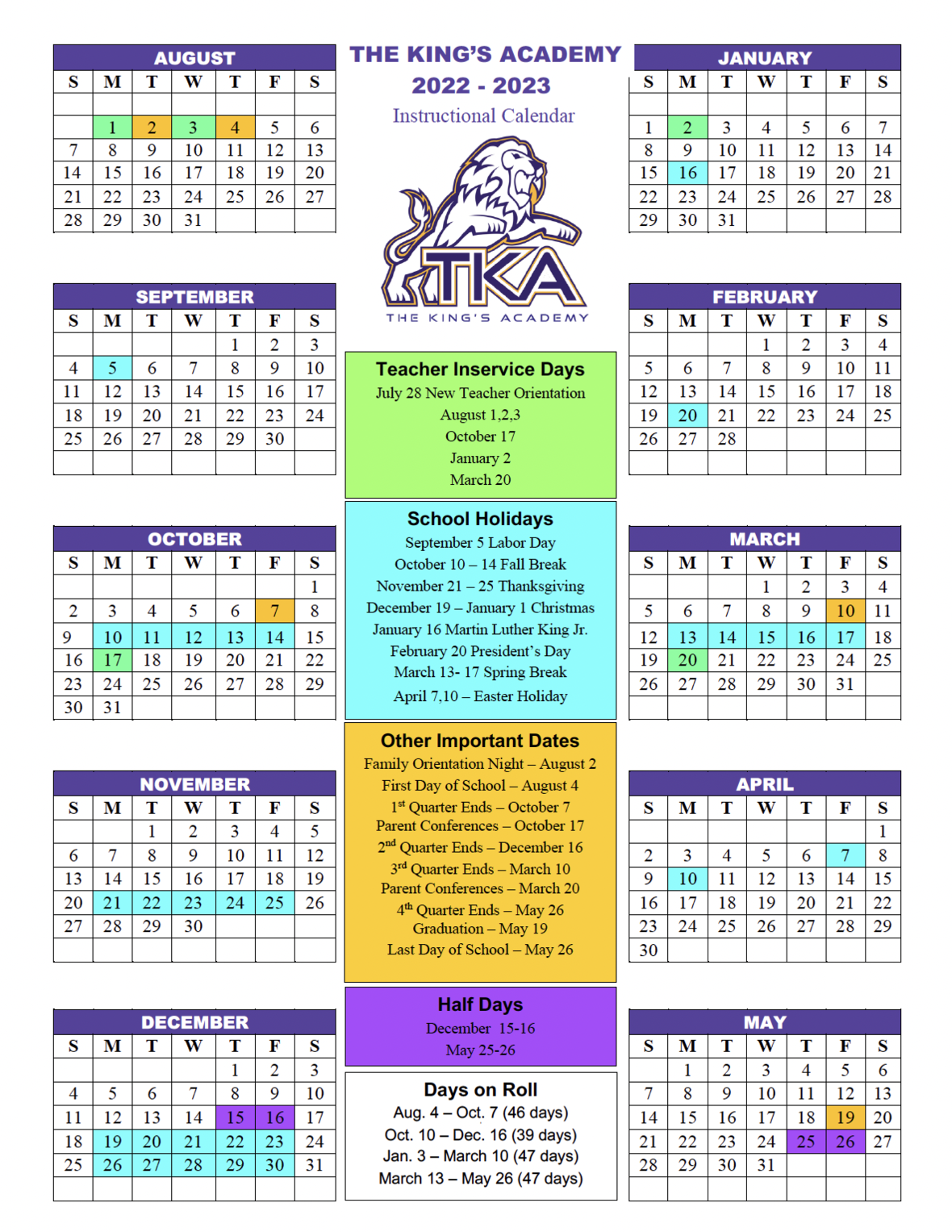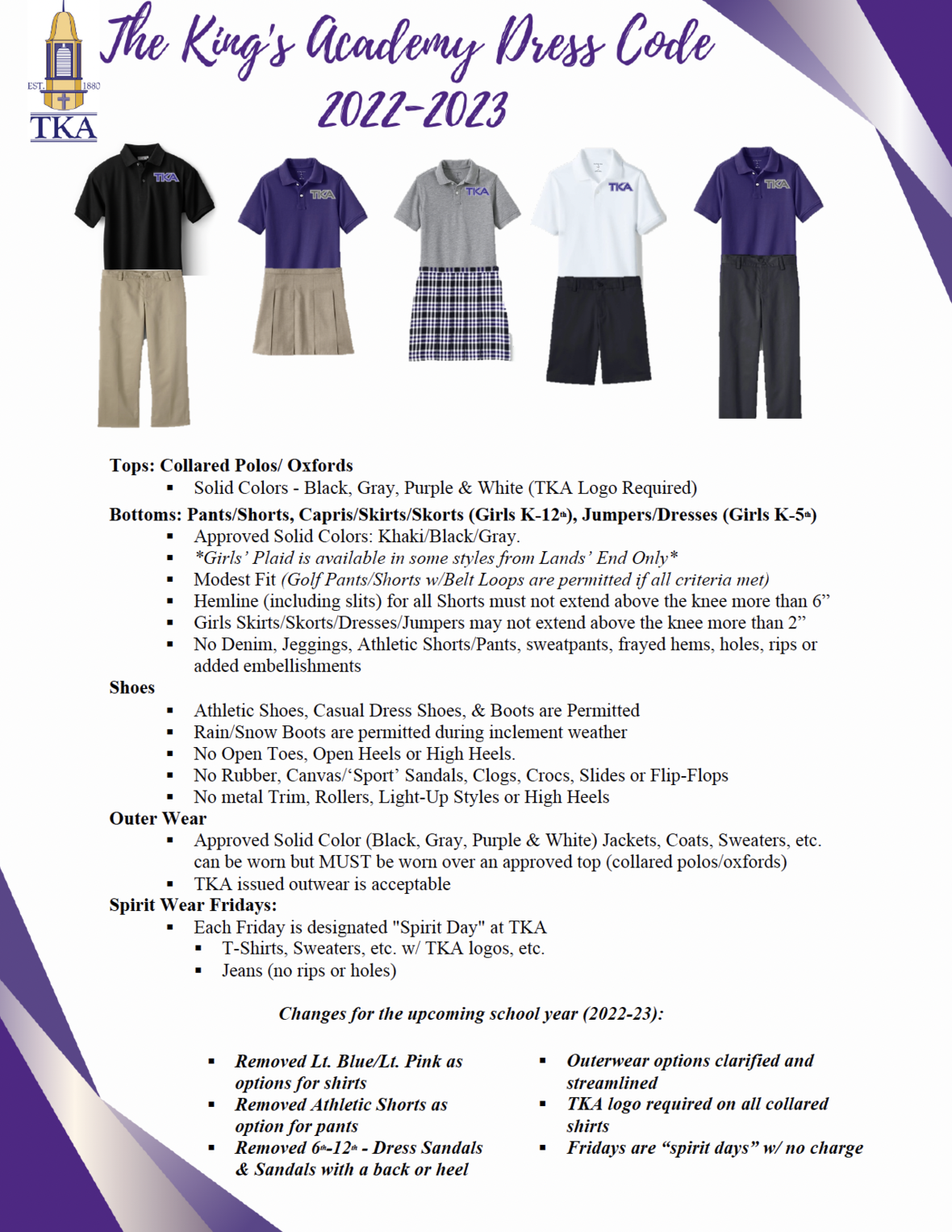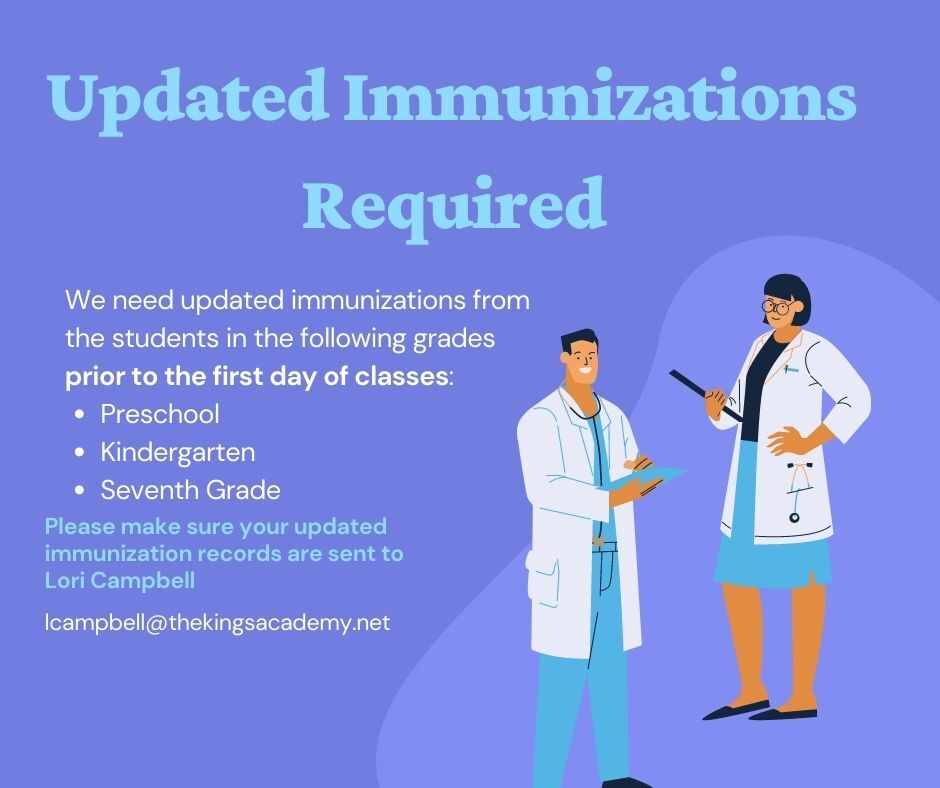table div table+table+table+table+table+table+table+table+table+table+table+table+table+table+table+table+table+table+table+table+table+table+table+table+table+table+table+table+table+table+table+table+table+table+table+table+table div table{width:100%;padding:0}table div table+table+table+table+table+table+table+table+table+table+table+table+table+table+table+table+table+table+table+table+table+table+table+table+table+table+table+table+table+table+table+table+table+table+table+table+table div table img{width:96.23%;padding:0;float:none}table div table+table+table+table+table+table+table+table+table+table+table+table+table+table+table+table+table+table+table+table+table+table+table+table+table+table+table+table+table+table+table+table+table+table+table+table+table div table td{width:100%;padding:0 1.88% 18px}/* styles */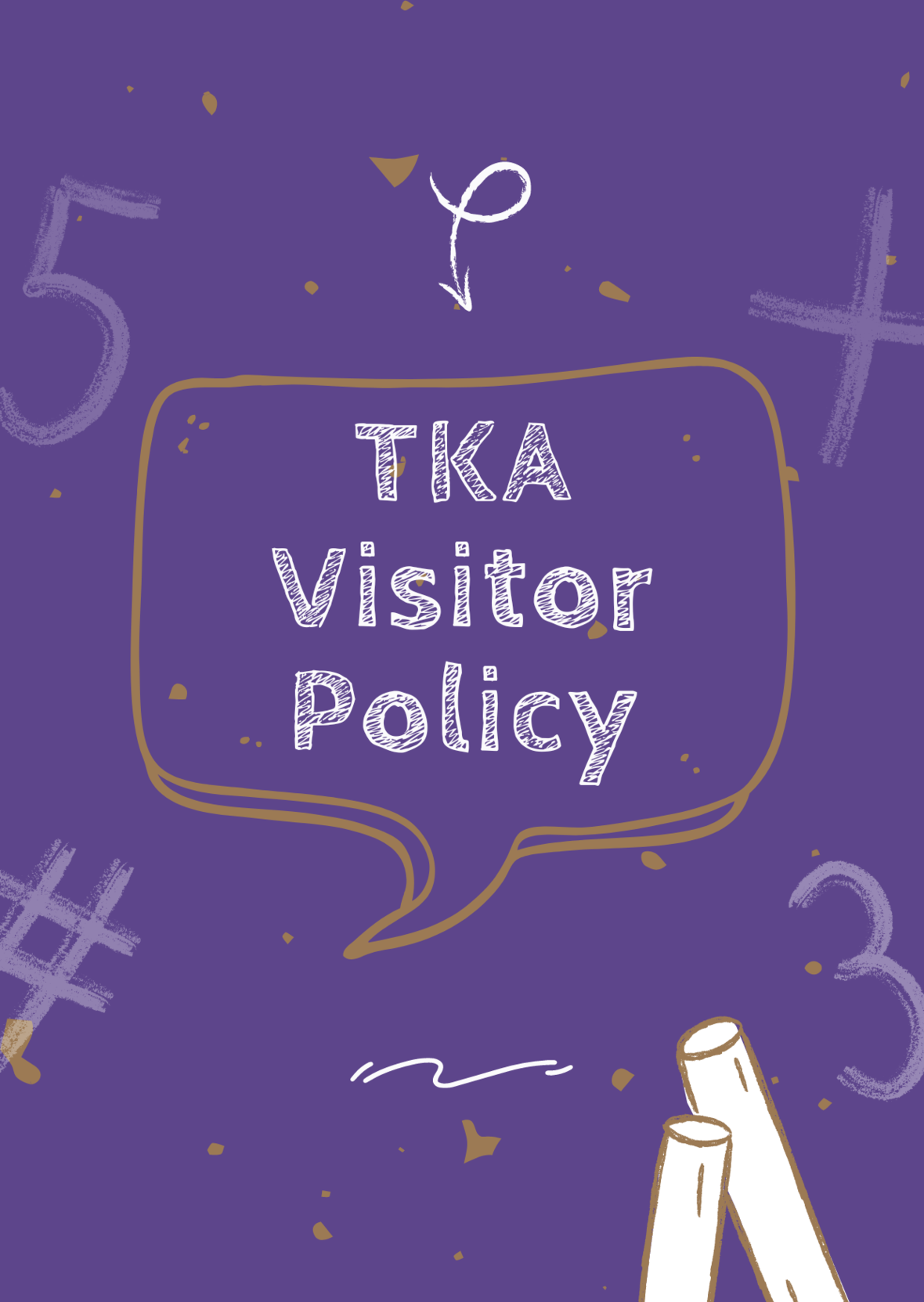# TKA Visitor Policy

In order to help maintain a safe and instructionally based learning environment for our students, all visitors for middle and high school must enter through the front door of the Anderson Building and sign in in the middle/high school office in order to receive a visitor's pass. All visitors for elementary must enter the front door of the Ogle Building in order to receive a visitor's pass. This pass should be visible at all times so that TKA faculty and staff know that you have checked in at the office.

Our doors are open to visitors, but please call ahead of time to schedule conferences with your child’s teachers. This will prevent interruptions to instruction and the loss of the valuable learning time of our students. Adults will not be allowed to wander the halls of the building or hold conferences with teachers during instructional times.

TKA family lunch visitors should follow the above procedure. Lunch tickets are \$5 each.

Students who are not enrolled at TKA are not permitted to visit TKA students during the school day, which includes lunch.

This policy has been developed in order to help maintain a safe and instruction focused environment at school each day. Any visitor who does not meet these expectations may be asked to leave school grounds.

 table div table+table+table+table+table+table+table+table+table+table+table+table+table+table+table+table+table+table+table+table+table+table+table+table+table+table+table+table+table+table+table+table+table+table+table+table+table+table+table div table{width:100%;padding:0}table div table+table+table+table+table+table+table+table+table+table+table+table+table+table+table+table+table+table+table+table+table+table+table+table+table+table+table+table+table+table+table+table+table+table+table+table+table+table+table div table img{width:96.23%;padding:0;float:none}table div table+table+table+table+table+table+table+table+table+table+table+table+table+table+table+table+table+table+table+table+table+table+table+table+table+table+table+table+table+table+table+table+table+table+table+table+table+table+table div table td{width:100%;padding:0 1.88% 18px}/* styles *//* styles */
 table div table+table+table+table+table+table+table+table+table+table+table+table+table+table+table+table+table+table+table+table+table+table+table+table+table+table+table+table+table+table+table+table+table+table+table+table+table+table+table+table+table+table div table{width:100%;padding:0}table div table+table+table+table+table+table+table+table+table+table+table+table+table+table+table+table+table+table+table+table+table+table+table+table+table+table+table+table+table+table+table+table+table+table+table+table+table+table+table+table+table+table div table img{width:96.23%;padding:0;float:none}table div table+table+table+table+table+table+table+table+table+table+table+table+table+table+table+table+table+table+table+table+table+table+table+table+table+table+table+table+table+table+table+table+table+table+table+table+table+table+table+table+table+table div table td{width:100%;padding:0 1.88% 18px}/* styles */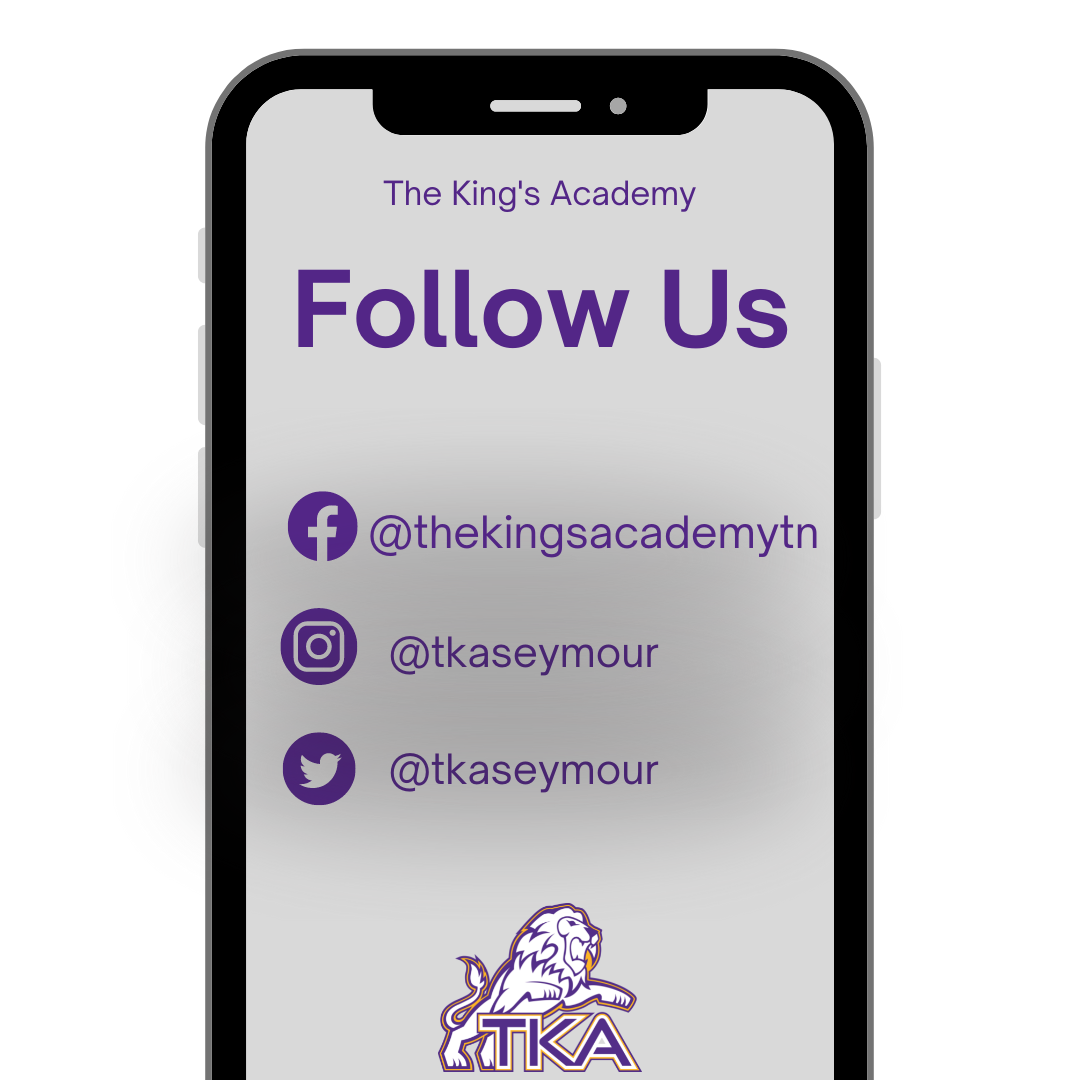table div table+table+table+table+table+table+table+table+table+table+table+table+table+table+table+table+table+table+table+table+table+table+table+table+table+table+table+table+table+table+table+table+table+table+table+table+table+table+table+table+table+table+table+table div table{width:100%;padding:0}table div table+table+table+table+table+table+table+table+table+table+table+table+table+table+table+table+table+table+table+table+table+table+table+table+table+table+table+table+table+table+table+table+table+table+table+table+table+table+table+table+table+table+table+table div table img{width:96.23%;padding:0;float:none}table div table+table+table+table+table+table+table+table+table+table+table+table+table+table+table+table+table+table+table+table+table+table+table+table+table+table+table+table+table+table+table+table+table+table+table+table+table+table+table+table+table+table+table+table div table td{width:100%;padding:0 1.88% 18px}/* styles *//* styles */ The King's Academy
 Like   Tweet